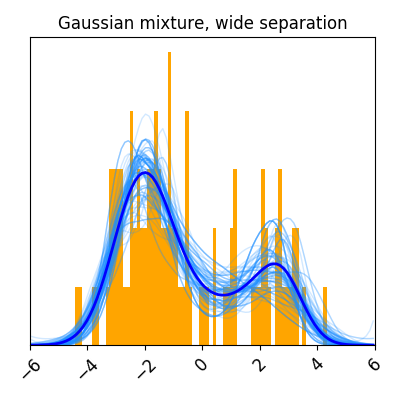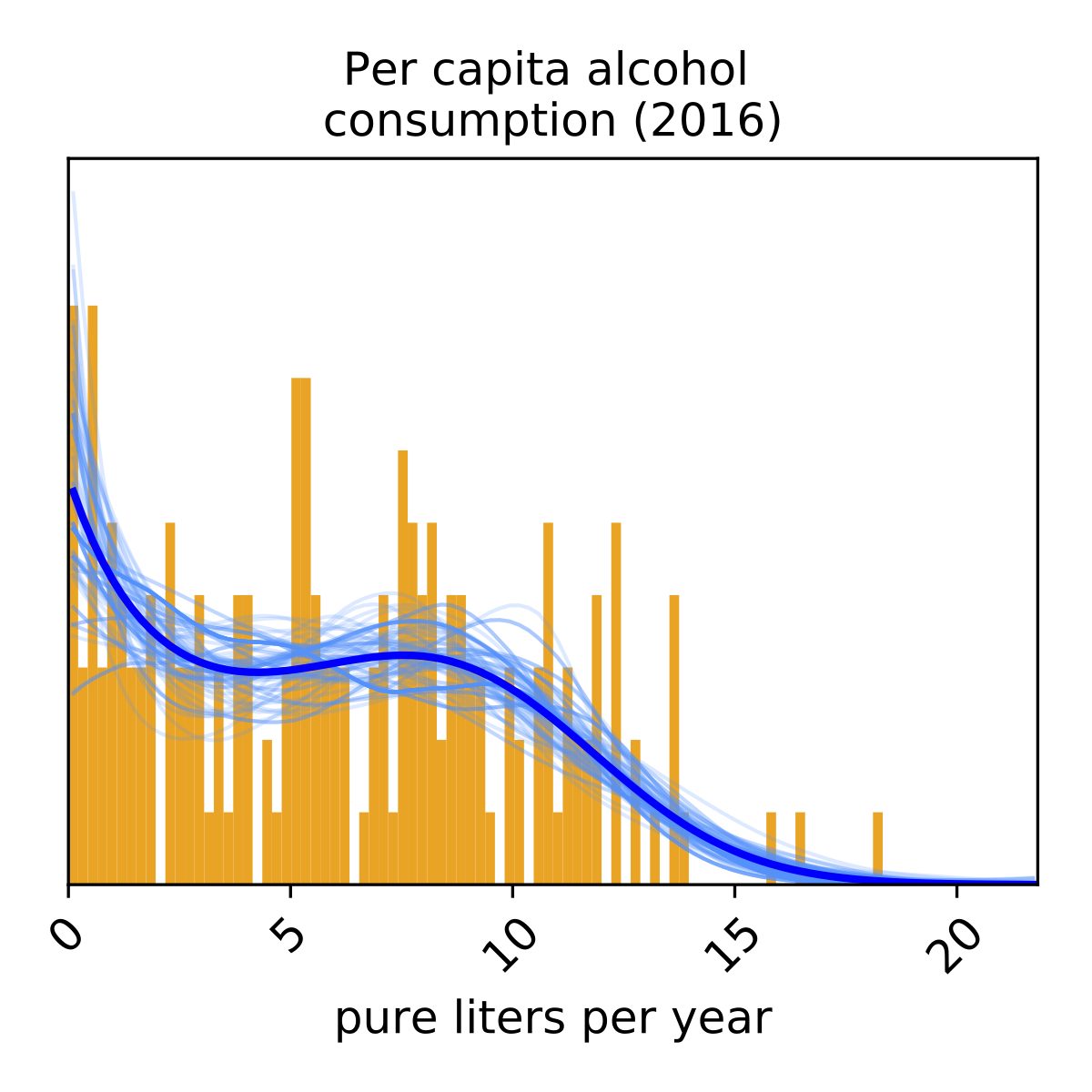# Examples¶

## Custom data¶```import numpy as np
import suftware as sw

# Generate random data
data = np.random.randn(100)

# Perform one-dimensional density estimation
density = sw.DensityEstimator(data)

# Plot results and save to file
density.plot(title='Gaussian')
```

## Simulated data¶```import suftware as sw

# Simulate data using a pre-specified distribution
dataset = sw.SimulatedDataset(distribution='wide', num_data_points=100)

# Perform one-dimensional density estimation
density = sw.DensityEstimator(dataset.data, bounding_box=dataset.bounding_box)

# Plot results and save to file
density.plot(title='Gaussian mixture, wide separation')
```

## Real data¶```import suftware as sw

# Retrieve data included with SUFTware
dataset = sw.ExampleDataset('who.alcohol_consumption')

# Perform one-dimensional density estimation
density = sw.DensityEstimator(dataset.data)

# Plot results and annotate with metadata
density.plot(title=dataset.description, xlabel=dataset.units)
```